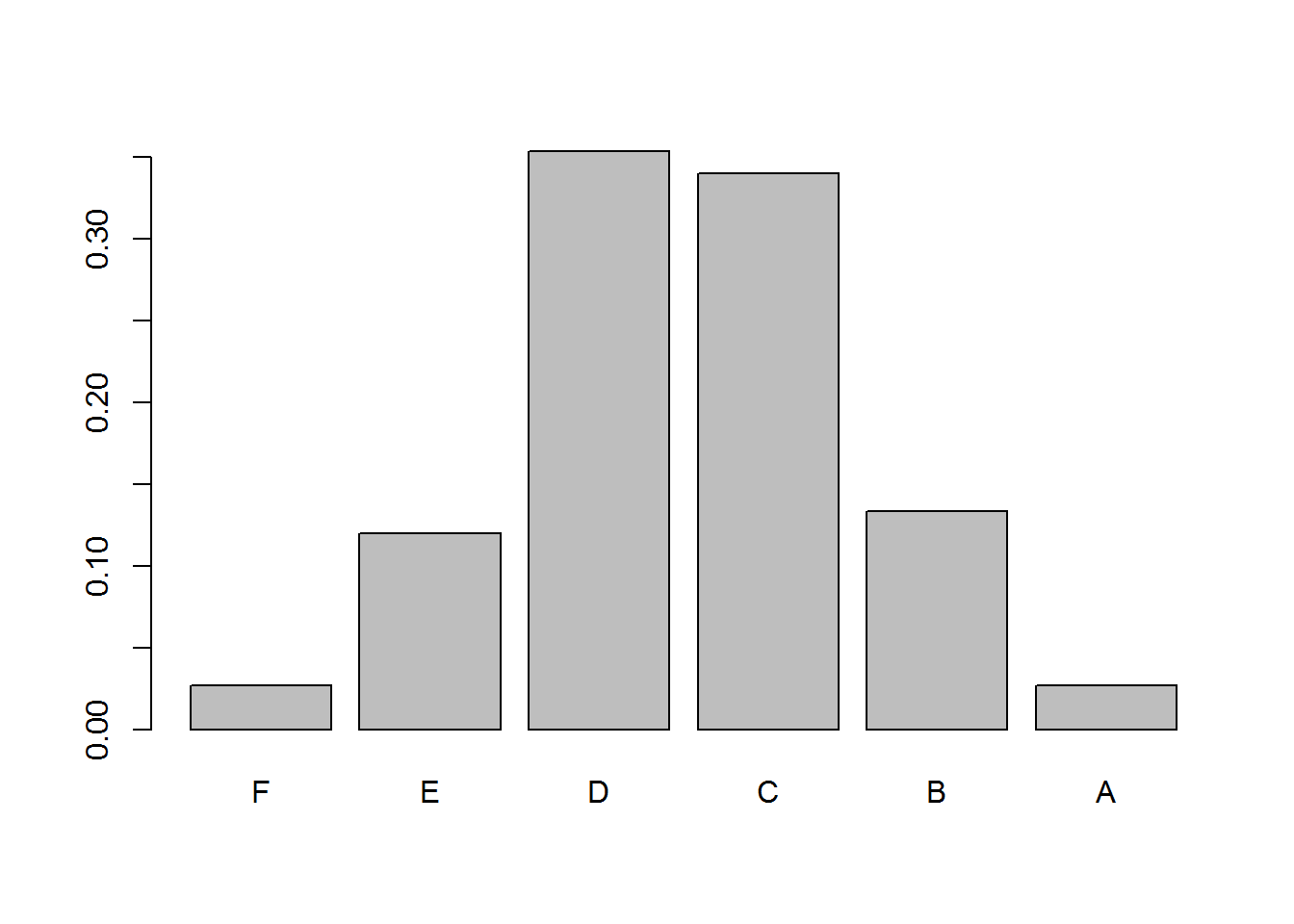### GRADING ON A CURVE DECOUPLES THE ACTUAL RAW SCORES FROM THE GRADE & MAKES IT A PEER-to-PEER SYSTEM:

#### The students:

n <- 150     # Number of students
x <- expand.grid(rep(list(c(LETTERS)), 2))
options <- do.call(paste0, x)
names   <- sample(options, size = n, replace = T)

#### The points - raw test scores:

points  <- c(sample(seq(40, 50, 0.1), 15, replace = T),
sample(seq(50, 60, 0.1), 46, replace = T),
sample(seq(60, 70, 0.1), 25, replace = T),
sample(seq(70, 80, 0.1), 47, replace = T),
sample(seq(80, 100, 0.1), 17, replace = T))

data <- data.frame(names, points)

hist(points, xlim=c(0,100), border = F, col = 'tan') # Not normal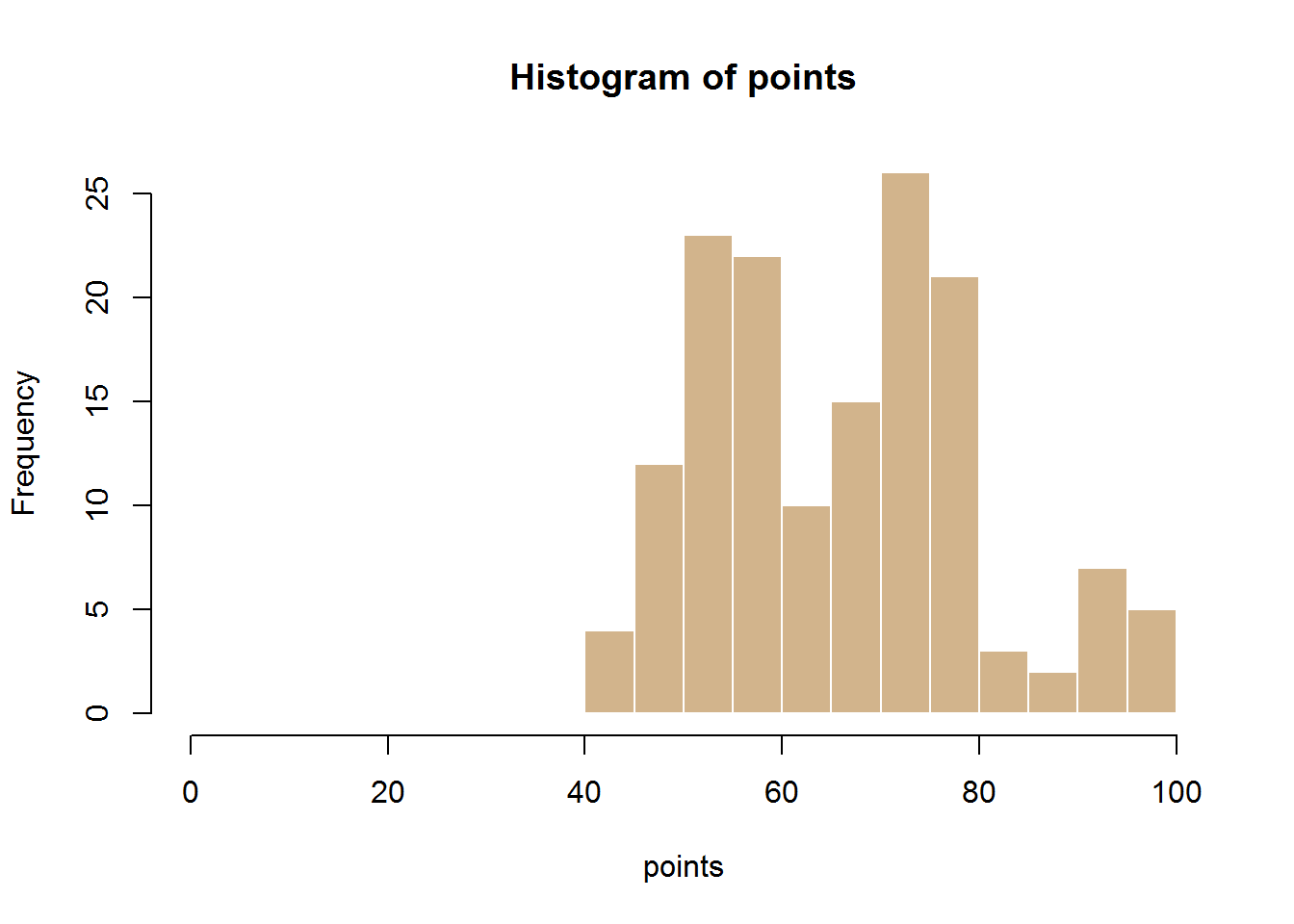shapiro.test(points)                    # ... with high degree of certainty...
##
##  Shapiro-Wilk normality test
##
## data:  points
## W = 0.9691, p-value = 0.001843
qqnorm(points)                                  # ...even visually.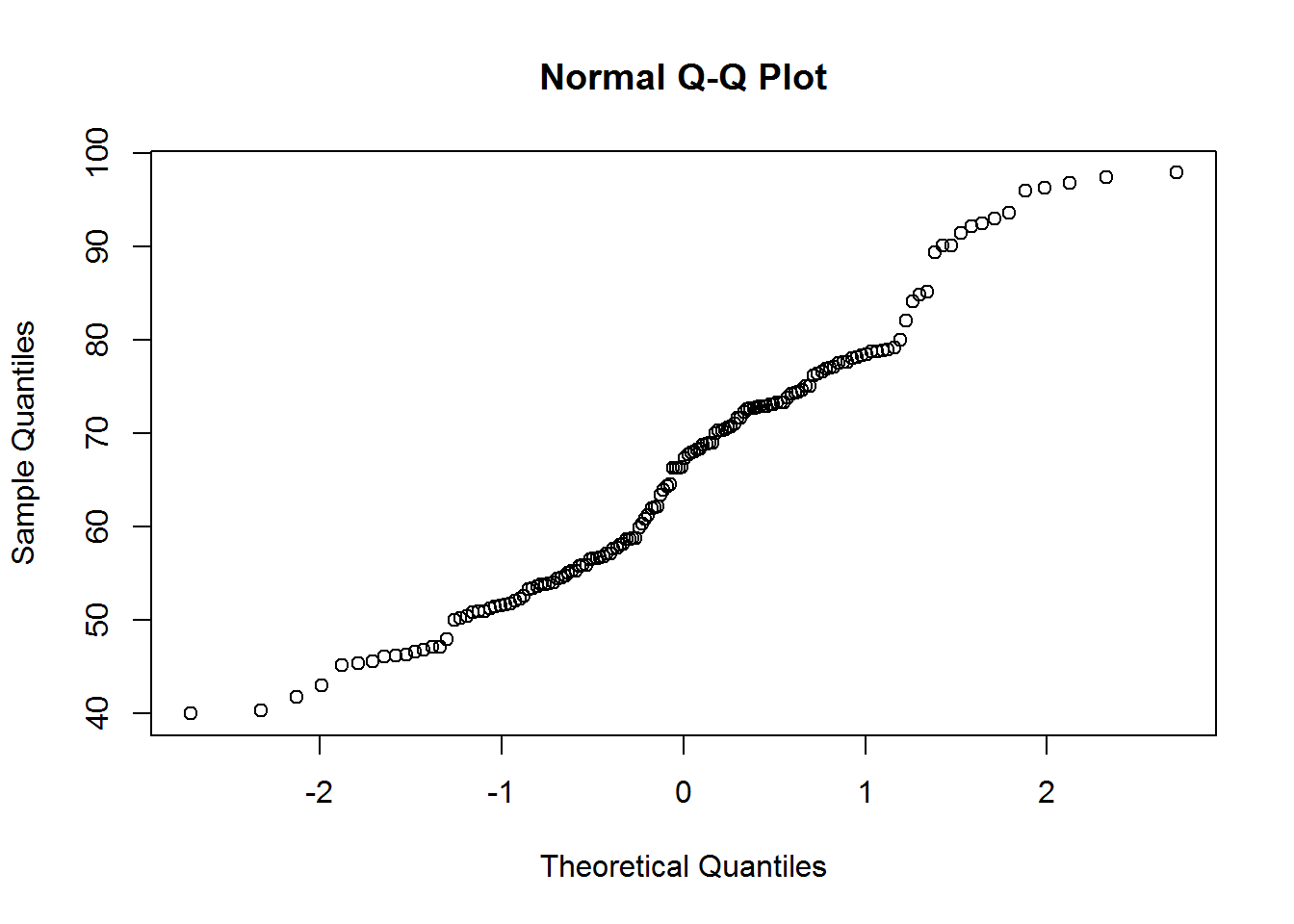cutoffs <- quantile(points, probs = pnorm(seq(-2,2))) # Select quantiles with frequency distr like SD of N(0,1)

data$final <- cut(data$points,
breaks=c(-Inf,cutoffs,+Inf),
labels=c(LETTERS[6:1])) # And we assign final grades based on these cutoffs

barplot(prop.table(table(data$final))) # Now they look normal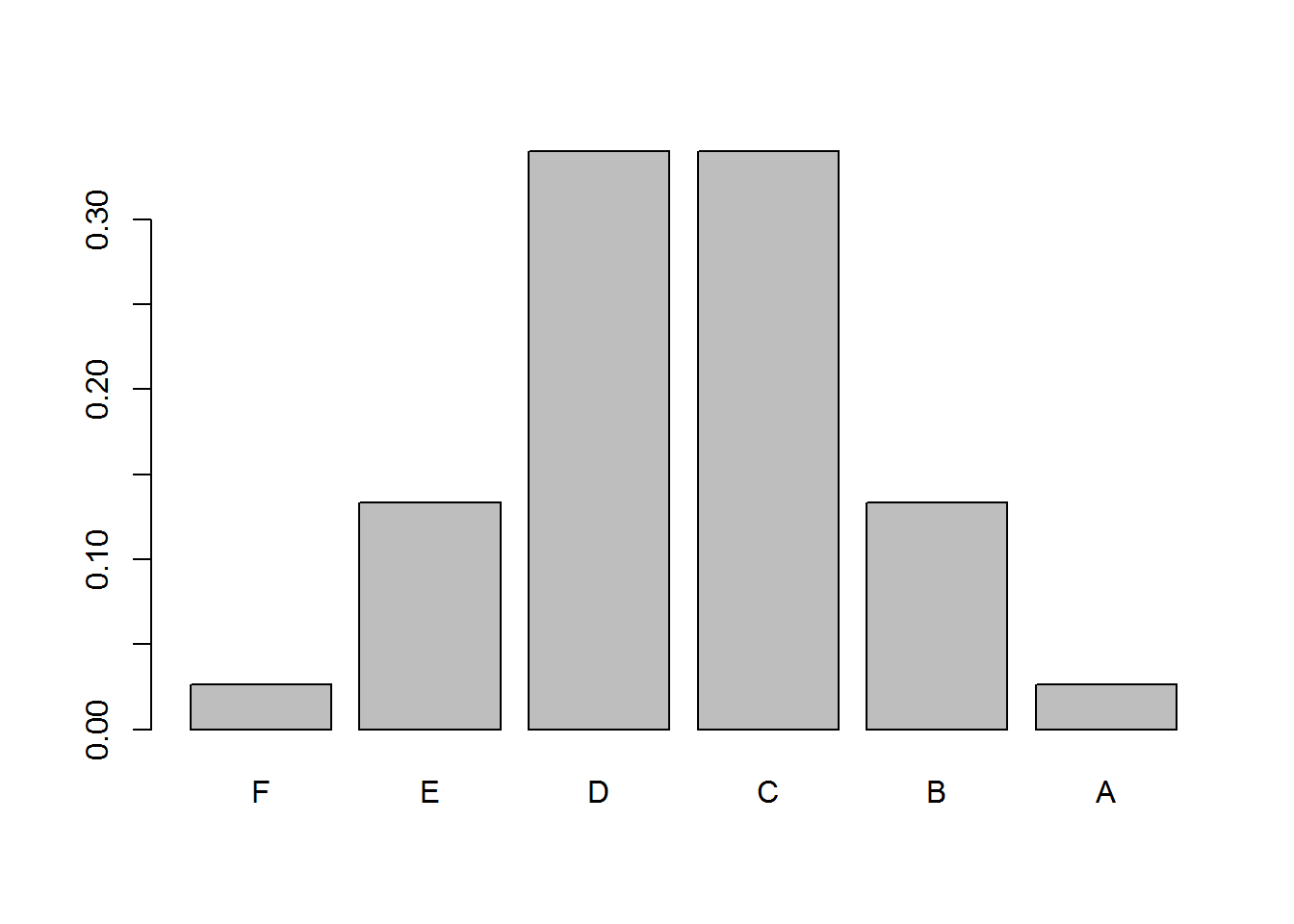(freq_distr <- prop.table(table(data$final))) # These is how the grades are distributed.
##
##          F          E          D          C          B          A
## 0.02666667 0.13333333 0.34000000 0.34000000 0.13333333 0.02666667
(Normal_distr <- c(pnorm(-2), pnorm(-1) - pnorm(-2), pnorm(0) - pnorm(-1),
pnorm(1) - pnorm(0), pnorm(2) - pnorm(1), pnorm(2, lower.tail = F))) 
##  0.02275013 0.13590512 0.34134475 0.34134475 0.13590512 0.02275013
# This is how the normal would have them.
chisq.test(freq_distr, p = Normal_distr) #And the GOF confirms normality.
## Warning in chisq.test(freq_distr, p = Normal_distr): Chi-squared
## approximation may be incorrect
##
##  Chi-squared test for given probabilities
##
## data:  freq_distr
## X-squared = 0.0015, df = 5, p-value = 1

Alternatively, the percentile can be given as the final grade, or used as an additional grade (percentile + ordinal score):

zscores <- scale(points) # Normalizes the points
mean(zscores); sd(zscores) #N(0,1)
##  2.490022e-16
##  1
hist(zscores, breaks = 18, border = F, col = 'tan') # The data distribution is normalized but not distributed normally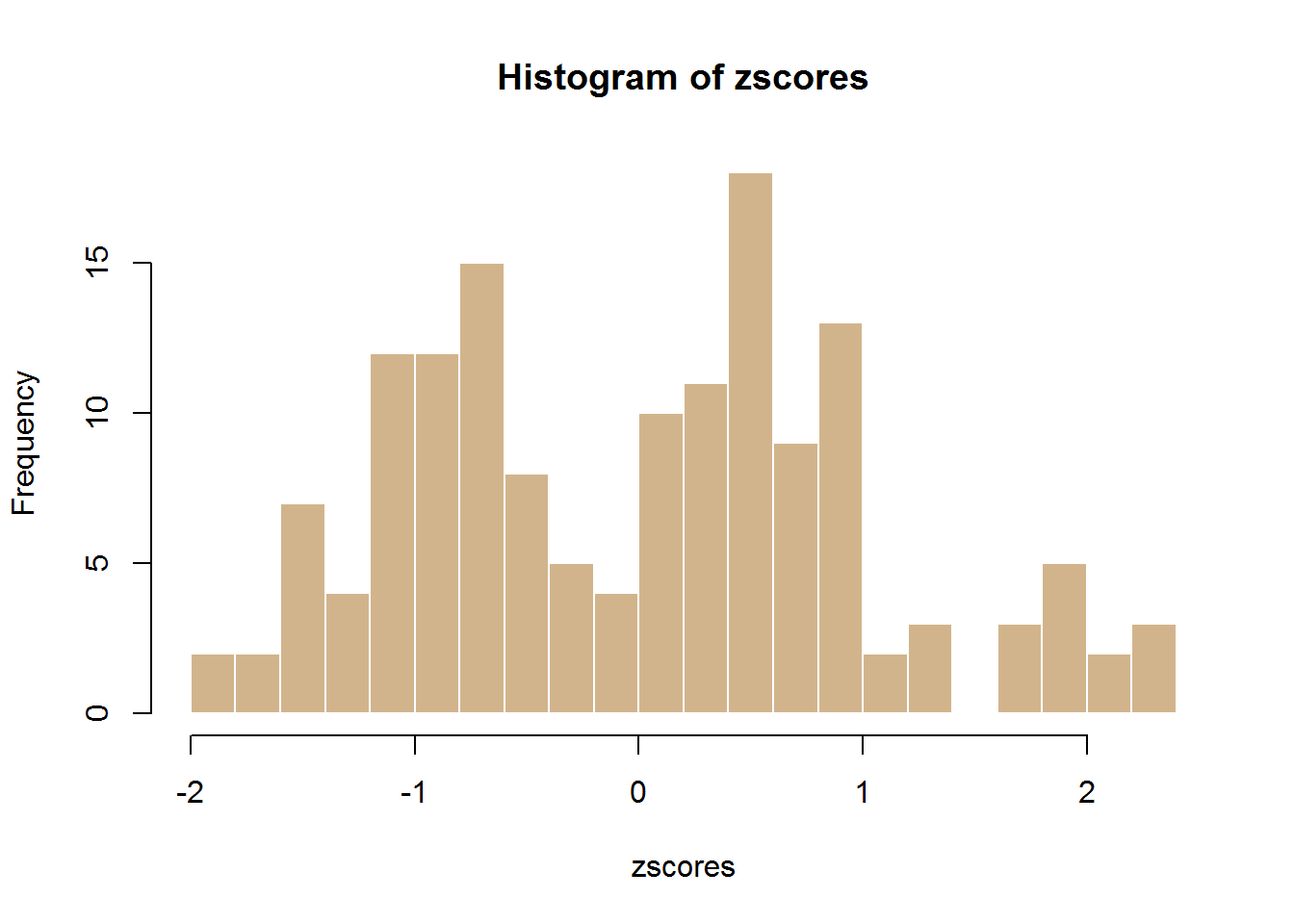percentiles <- pnorm(zscores) * 100
plot(percentiles, pch = 20, col = 2)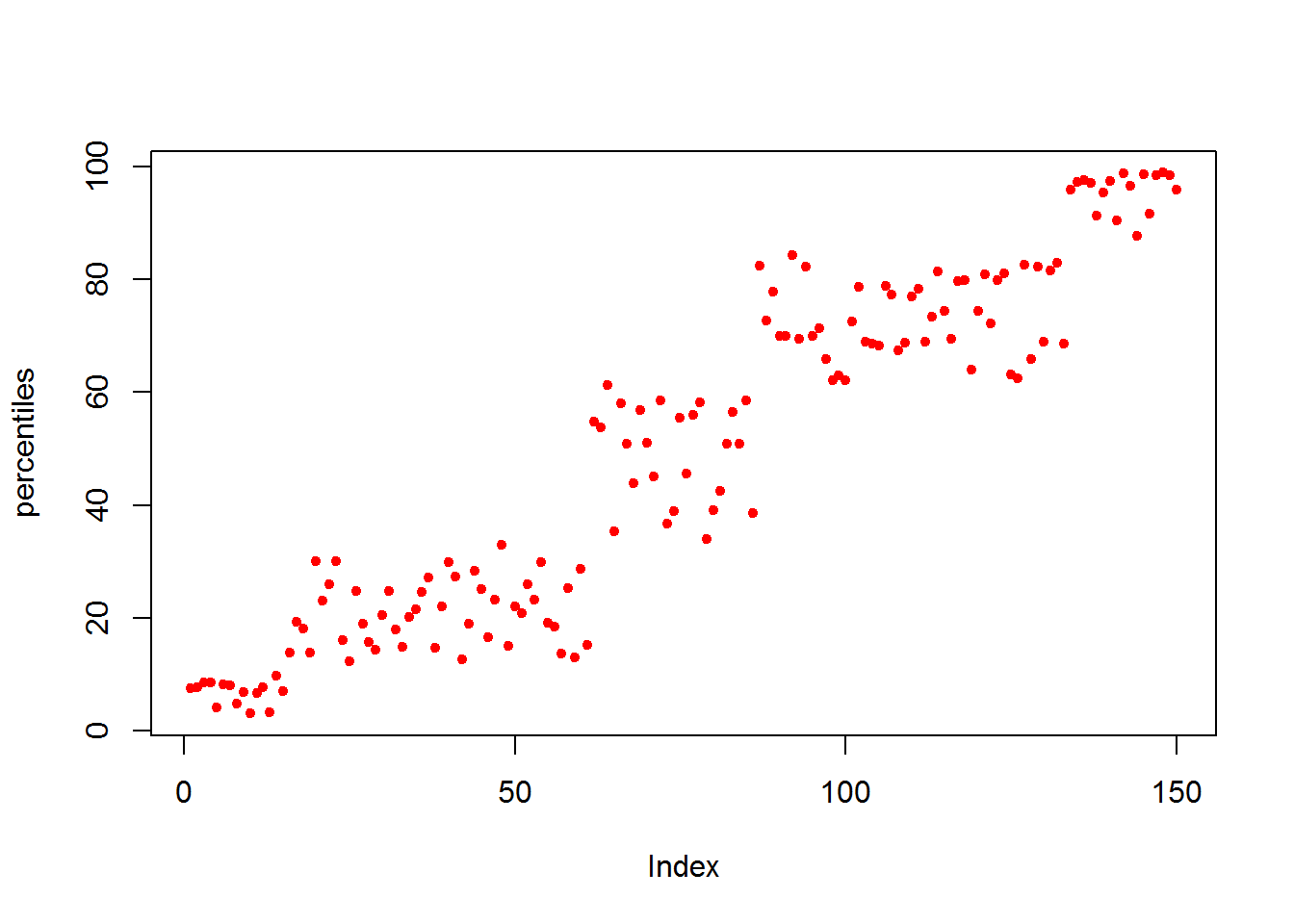At this point we can assign the percentiles as the final grade:

data$percentiles <- round(percentiles) tail(data) ## names points final percentiles ## 145 LV 96.8 A 99 ## 146 MP 85.2 B 92 ## 147 SQ 96.0 B 98 ## 148 VC 97.9 A 99 ## 149 DQ 96.3 A 99 ## 150 KV 90.1 B 96 Or we can assign grades based on percentiles as we did with “points”: cutoffs <- quantile(percentiles, probs = pnorm(seq(-2,2))) data$alternative <- cut(data$percentiles, breaks=c(-Inf,cutoffs,+Inf), labels=c(LETTERS[6:1])) # And we assign final grades based on these cutoffs tail(data) ## names points final percentiles alternative ## 145 LV 96.8 A 99 A ## 146 MP 85.2 B 92 B ## 147 SQ 96.0 B 98 B ## 148 VC 97.9 A 99 A ## 149 DQ 96.3 A 99 A ## 150 KV 90.1 B 96 B barplot(prop.table(table(data$alternative)))